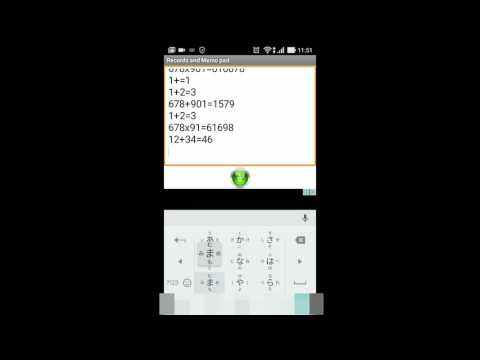# Notes and calculator with history functionEveryone
52Equipped saving of calculation history and notepad function
Smart design based on actual calculator.
Calculation of memory constants How to use calculator functions such as exponentiation and manual screen on which practical mathematical formulas can be seen

Save your calculation history and view past equations at any time.
You can save what you have entered even after exiting the app.
You can freely edit, copy, paste, and erase your calculation history.
As you can also freely edit, and enter text on the calculation history screen, it may also be used to take notes.

•This app does not fall short when compared to Calculator Plus, or other classic apps with practical-use functions.
You can use it with confidence in not only business or at work, but also in studying for tests and other kinds of learning.

•There is no limit to how many numbers can be entered and shown.
Utilizing screen optimization technology, very large numbers can be input and calculated.

•Calculator manual available
You can view a manual which shows how to use practical mathematical expressions with calculator functions like memory, constant calculations, exponents, etc.

Functional 4 color key
It is color-coded according to function, and visibility at the time of key input is improved.

Calculation status display

You can check calculation of arithmetic operation and use status of memory function by display.

① Calculator manual
Memory constant calculations You can view manuals that show how to use the calculator functions such as the exponentiation.

② useful formulas, practical formulas
You can browse 99 mathematical expressions useful for business, daily life, technical work, sports etc.
If you enter a number, you can see the value of that formula.

③ Unit converter
Weight, Distance, Area, Capacity, Temperature, Speed, Power, Pressure, Work, Output, Angle, Heat Amount, Radiation, Magnetic force, Equipped with unit conversion function, unit conversion is possible by entering numbers.

• Function List
•% (Percent) calculations ( ×% ÷% -% +% ) can be used in consumption tax calculations.
•Constant calculations ( × ÷ - + ) Press the Operator button twice
•Exponents, press the x button twice Example: 5 xx = 25
•Built-in memory function
•Memory key (M+, M-)
•00-key (for hundreds)
•Backspace (→) key
•+/- (sign change) key

•Other Functions
Sound can be turned ON or OFF
Vibration can be turned ON or OFF

Calculate percentage (percent)

M [×] n [%] Find n% of m
M [÷] n [%] Find what percentage of n is m

Built-in memory function

"Memory (MEMORY)" is used when you want to temporarily memorize entered numbers.

"M +" (Memory Plus): Add the number displayed on the calculator to the memory.
"M -" (memory minus): Subtract the number displayed on the calculator from the memory.
"MR" (Memory Recall): Press to recall the memory contents.
"MC" (Memory clear): Press to clear the memory contents (0)

Exponentiation (multiplication) × press twice Example: 5 xx = 25

Constant calculation (× ÷ - +) Operator button Press twic

Other functions
Vibration ON, OFF possible

You were probably using a difficult-to-operate function calculator. Your friend was probably using the seemingly-convenient "Calculator Hide App" and "Calculator Vault." But, wait a minute. This Pure Gold Calculator definitely doesn't seem to be compatible with those. However, the unique UI and user-friendliness will leave you unable to ignore it. It certainly lacks features like the loan calculator and the car calculator. However, with a little creativity those calculations can be performed as well. Since it has the convenient Calculation History available, getting used to the app's functionality allows you to perform very complicated calculations with ease.
Collapse

Review Policy
4.1
52 total
5
4
3
2
1

## What's New

Bug fixed 19/01/29
Collapse

Updated
January 29, 2019
Size
6.1M
Installs
10,000+
Current Version
14.0
Requires Android
4.0 and up
Content Rating
Everyone
Permissions
Offered By
ATPC Japan Co.,Ltd.
Developer